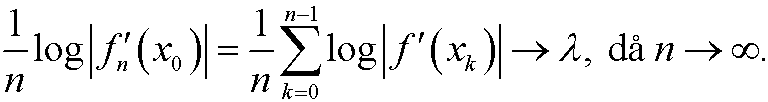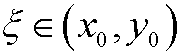3. The Lyapunov exponent.

We define fn(x) for a given function f(x) and a given starting value x0 byBy the chain rule we then have thatBy taking logarithms and dividing by n on both sides we getThis convergence can be proved by using a particular mathematical technique. The limitis called the Lyapunov exponent of the dynamical system.

Now suppose that we have two (close) starting values x0 and y0. By using the mean value theorem we see thatsinceandThe conclusion is that

a) ifwe have thatthat is, the system is not sensitive to the starting value.

b) ifthendiverges to infinity with exponential growth.